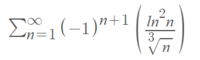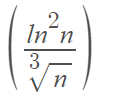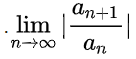# Does it converges?

#### sinos

##### New member
Hello,
I have two question:
Firstly,
I got this problem:I should determine weather the series converges.
I thought about using leibinz test but I don't know how to prove thatis monotonlly decreasing.

The second qeustion I would like to ask is, if I don't know about a series if its positive, can I use d'almbert ratio test in this way:and if for example for the none positive series I show in this way that the series diverges is this a legit proof?
Thank you!

#### Attachments

• 3.4 KB Views: 0

#### pka

##### Elite Member
I have two question: Firstly,
I got this problem: View attachment 12995

I should determine weather the series converges.
I thought about using leibinz test but I don't know how to prove that View attachment 12996
is monotonlly decreasing.
The second qeustion I would like to ask is, if I don't know about a series if its positive, can I use d'almbert ratio test in this way:Consider the function $$\displaystyle f(x)=\frac{\log^2(x)}{x^{1/3}}$$ then $$\displaystyle f'(x)=-\frac{(\log(x)-6)\log(x)}{3x^{4/3}}$$ SEE HERE
Can you see that the derivative is negative if $$\displaystyle x>e^6~?$$
Thus apply the alternating series test. Post your results. So we can proceed.

#### sinos

##### New member
Consider the function $$\displaystyle f(x)=\frac{\log^2(x)}{x^{1/3}}$$ then $$\displaystyle f'(x)=-\frac{(\log(x)-6)\log(x)}{3x^{4/3}}$$ SEE HERE
Can you see that the derivative is negative if $$\displaystyle x>e^6~?$$
Thus apply the alternating series test. Post your results. So we can proceed.

Hi,
Why did you use log instead of lan? (I know lan is a private case of log but still I don't understand)

thank you

#### pka

##### Elite Member
Hi,
Why did you use log instead of lan? (I know lan is a private case of log but still I don't understand)

thank you
It is current practice to use $$\displaystyle \log(n)$$ for the so-called natural logarithm.

#### lookagain

##### Elite Member
Hi,
Why did you use log instead of lan? (I know lan is a private case of log but still I don't understand)

thank you
If you got your problem for a course/from an instructor, then you answer it back with the same "ln" notation.

And, no, pka is not correct about it being (exclusive) current practice. His statement comes from his bias.
Your own question is testament to that. It is in mixed usage today.

•topsquark, sinos and Dr.Peterson

#### sinos

##### New member
okay.
Thank you so much!